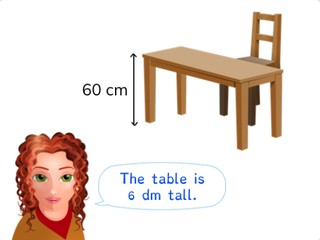Converting cm and dm

# Converting cm and dm

Students learn to convert cm and dm.

No account needed.8,000 schools use Gynzy92,000 teachers use Gynzy1,600,000 students use Gynzy

## General

The students learn to convert centimeters to decimeters and vice versa.

## Standards

CCSS.Math.Content.4.MD.A.1

## Learning objective

Students will be able to convert centimeters (cm) to decimeters (dm) and vice versa.

## Introduction

On the interactive whiteboard there are a number of measurements of an object. The students must determine if these are in centimeters or meters. You can drag the cm and m to the lines.

## Instruction

You explain using a diagram of the metric system that when you want to convert centimeters to decimeters, you take one jump to the left. You explain that you therefore divide the centimeters by ten, because you take one jump dividing by ten. You then show this in the exercises in which you convert the centimeters to decimeters. After this you explain that when you don't have an exact number of decimeters, you will have a number of centimeters left over. By first seeing how many decimeters you have you can see how many centimeters are left over. You have the students drag the numbers to the correct boxes. You also explain that when you want to convert decimeters to centimeters, you take one jump to the right and therefore you multiply by ten. You show this by converting various lengths in decimeters to centimeters. Next you explain that when you don't have an exact amount of decimeters, you first convert the decimeters to centimeters and then add on the extra centimeters. The students drag the numbers to the correct boxes. Finally you have the students solve story problems. For this, explain what steps you follow when solving this type of story problem.

To check whether students can convert centimeters to decimeters and vice versa, you can ask the following questions:
- How many centimeters is one decimeter?
- What do you do when you convert centimeters to decimeters?
- What do you do when you convert decimeters to centimeters?

## Quiz

The students test their understanding of converting centimeters to decimeters, and vice versa, through ten exercises. In some of these the students must convert from centimeters to decimeters, and the others they must convert decimeters to centimeters. Some of the exercises are story problems.

## Closing

Repeat the learning goal and its importance. Next there is an amount of decimeters with centimeters on the whiteboard. Under this are three answers. The students must determine which amount is correct. When you erase the answer that the students state and it turns green, the answer is correct. If it is incorrect, the answer turns red. After this there is an amount in centimeters and the students must convert this to decimeters.

## Teaching tips

When students have difficulty with this learning goal, you can give them a diagram of the metric system, in which you only have decimeters and centimeters with an arrow for ÷10 and an arrow for ×10.

## Instruction materials

You may print out a diagram of the metric system.

### The online teaching platform for interactive whiteboards and displays in schools

• Save time building lessons

• Manage the classroom more efficiently

• Increase student engagement